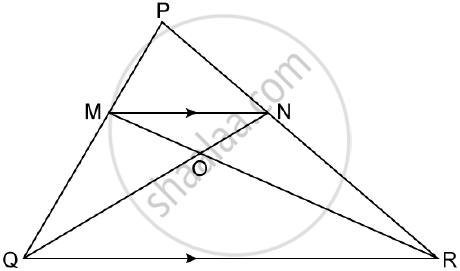Share

Books Shortlist

# In δPqr, Mn is Parallel to Qr and (Pm)/(Mq) = 2/3 and Find (Mn)/(Qr) and Prove that Triangle Omn and Triangle Orq Are Similar - ICSE Class 10 - Mathematics

#### Question

In ΔPQR, MN is parallel to QR and (PM)/(MQ) = 2/3

1) Find (MN)/(QR)

2) Prove that ΔOMN and ΔORQ are similar.

3) Find, Area of ΔOMN : Area of ΔORQ#### Solution

In ΔPMN and ΔPQR, MN || QR

=> angle PMN =anglePQR   (alternate angles)

=> angle PNM = anglePRQ  (alternate angles)

=> ΔPMN ~ ΔPQR (AA postulate)

= (PM)/(PQ) = (MN)/(QR)

=> 2/5 = (MN)/(QR)    [(PM)/(MQ) = 2/3 => (PM)/(PQ) = 2/5]

2) In ΔOMN and ΔORQ,

angleOMN = angleORQ   (alternate angles)

angleMNO = angleOQR    (alternate angles)

=> ΔOMN ~ ΔORQ  (AA postulate)

3) "Area of ΔOMN"/"Area of ΔORQ" = (MN)/(RQ) = 2/5

Is there an error in this question or solution?

#### APPEARS IN

2017-2018 (March) (with solutions)
Question 9.2 | 3.00 marks

#### Video TutorialsVIEW ALL 

Solution In δPqr, Mn is Parallel to Qr and (Pm)/(Mq) = 2/3 and Find (Mn)/(Qr) and Prove that Triangle Omn and Triangle Orq Are Similar Concept: Similarity of Triangles.
S